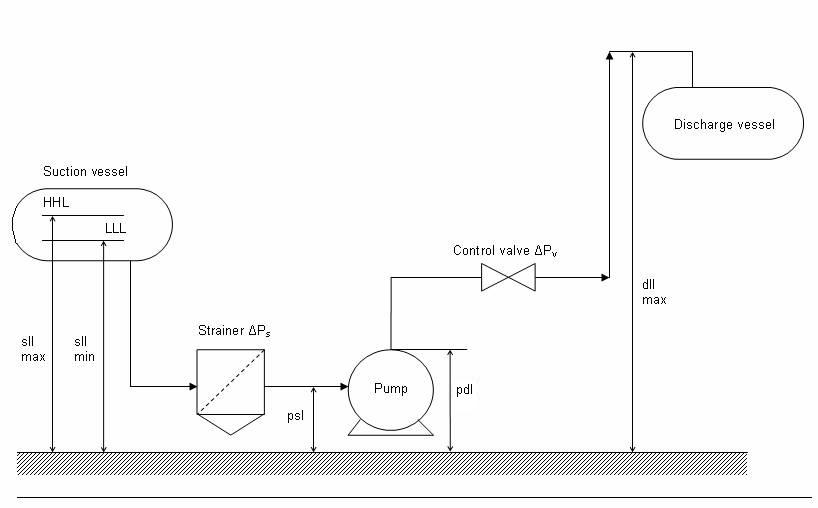# Pump sizing calculator

Consider a system such as shown in the following schematic. This is a basic or most common system where pumps are used for fluid transportation.

This page provides a basic calculation tool for sizing of pumps for a system such as given in the schematic.For the schematic given above,

 FLOW INPUTS W - Mass Flow rate kg/hr ρ - Fluid density kg/m3 μ - Fluid viscosity cP T - Fluid temperature 0C Pv - Vapour pressure at T bara Expected pump efficiency % Expected motor efficiency % SUCTION SIDE INPUTS Psv op (suction vessel operating Pressure) barg Sll min (Minimum suction side liquid level- refer the schematic) m ΔPsuct (Pressure loss across the straight suction line) bar ΔPs (Pressure loss across the strainer / filter) bar ΔPsother (Additional pressure loss on the suction side from fitting etc.) bar Psl (Pump suction nozzle level – refer the schematic) m DISCHARGE SIDE INPUTS Pdl (Pump discharge nozzle level – refer the schematic) m Pdv op (discharge vessel operating Pressure) barg ΔPdisch (Pressure loss across the straight discharge line) bar ΔPcv (Pressure loss across the control valve) bar ΔPdother (Additional pressure loss on the discharge side from fitting etc.) bar dll max (Maximum liquid level required to be attained on the discharge side – refer the schematic) m RESULTS NPSHa (available) m Suction pressure barg Dischrge pressure (required) barg Differential pressure (required) bar Shaft Power (required) kW Motor Power (required) kW

This pump sizer tool will output the shaft power and motor power requirement for your given service, flow rate and differential head required across the pump.